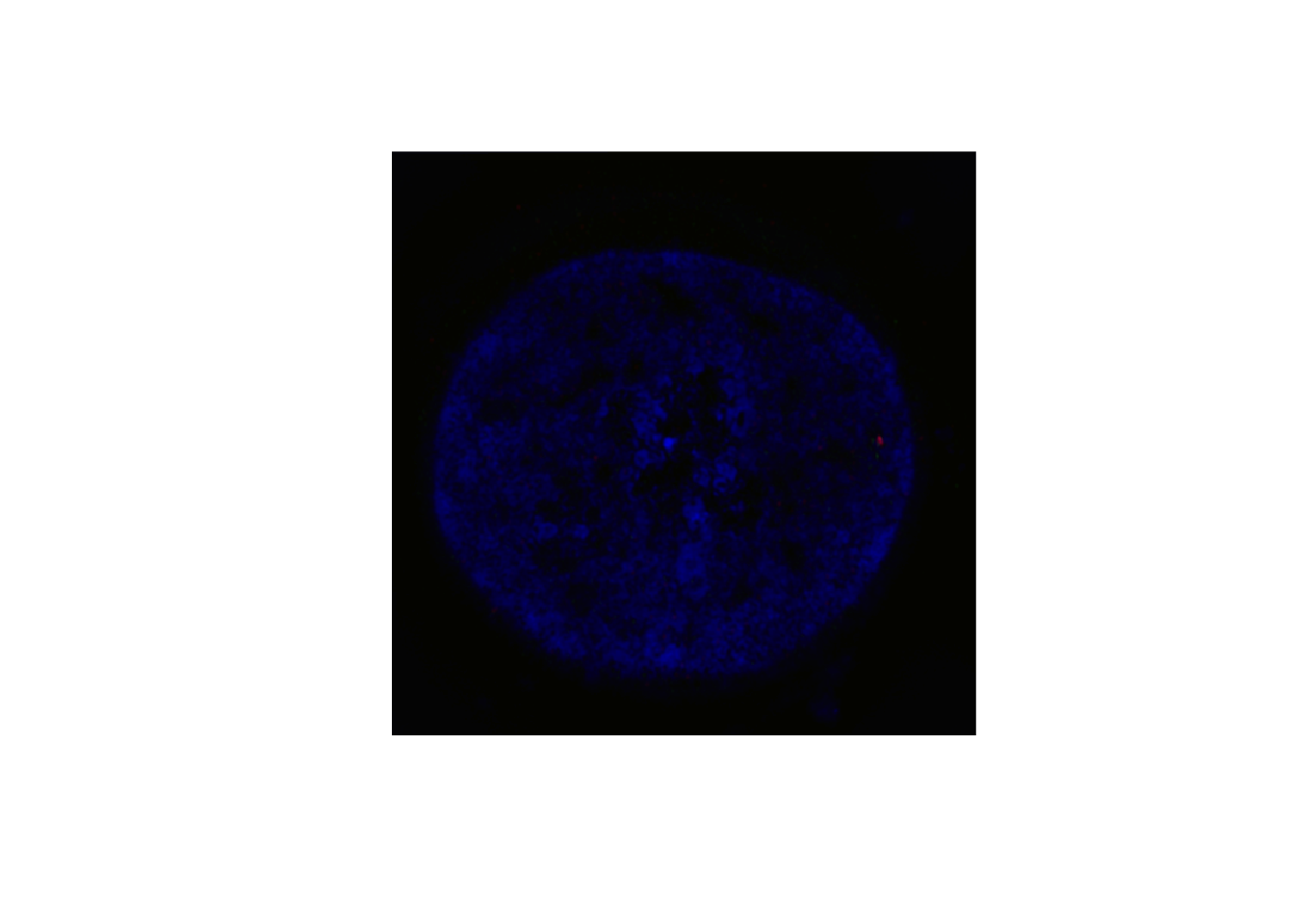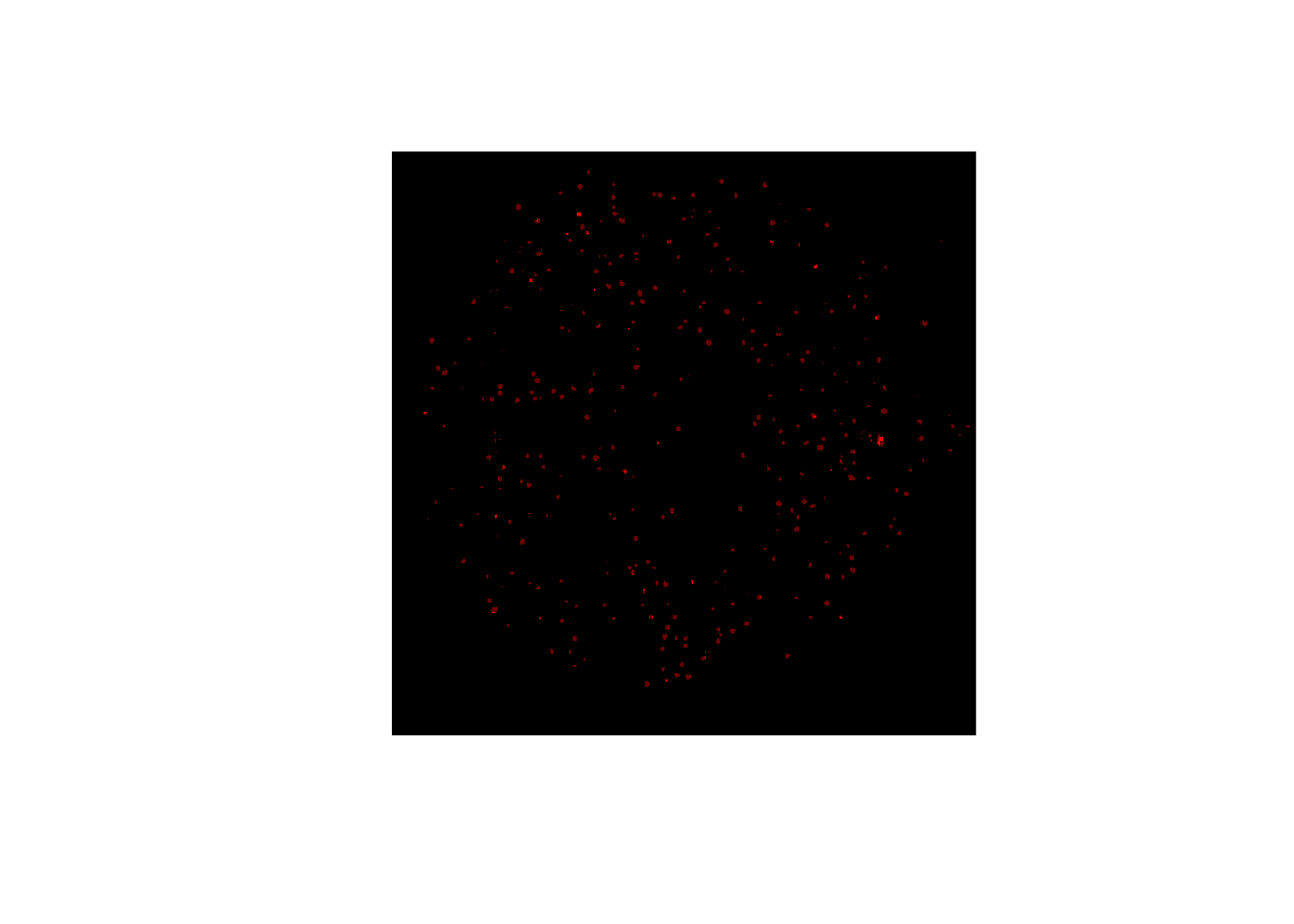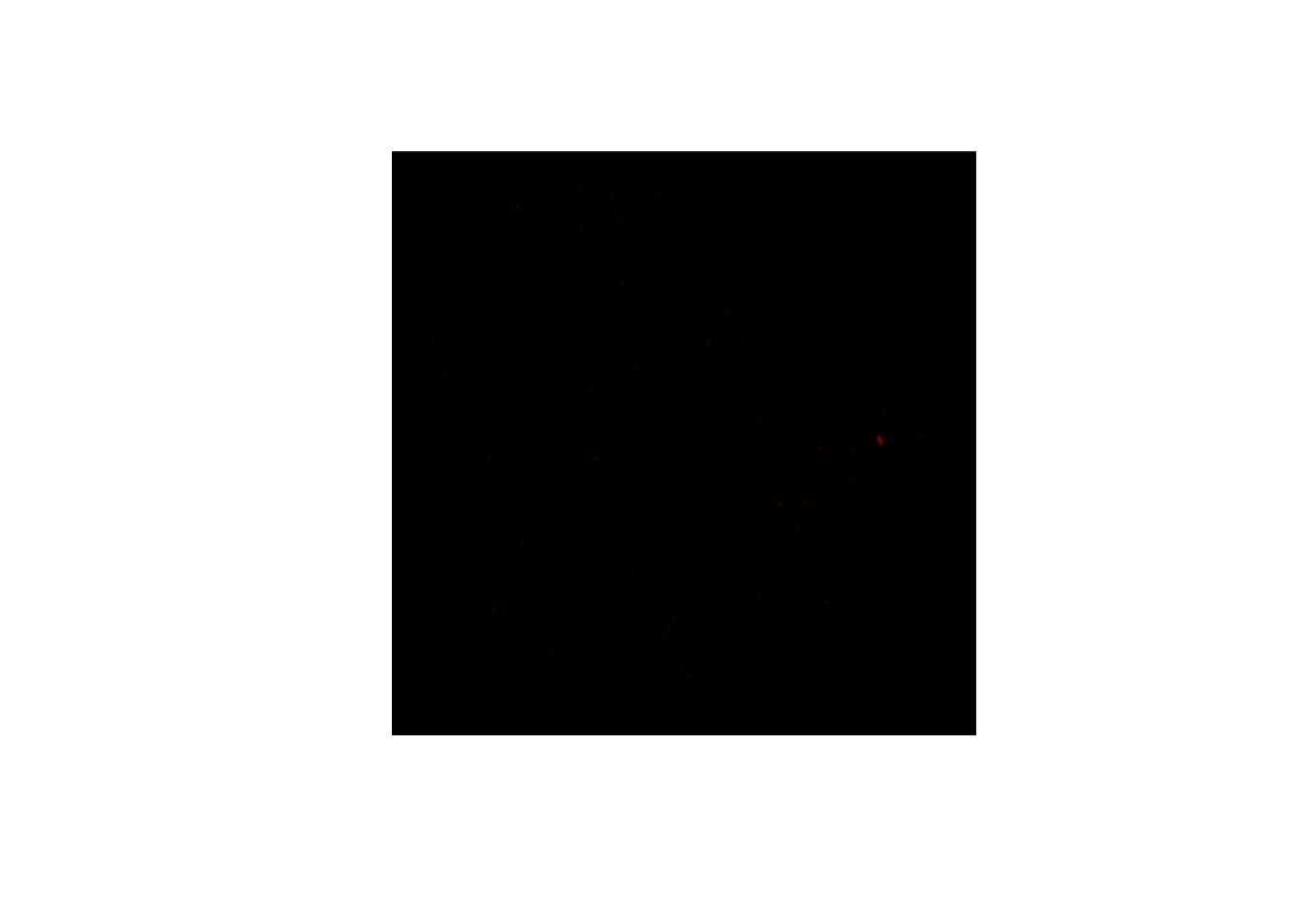# Read, write and plot TIFFs

#### 2020-05-29

``library(bioimagetools)``

## Read and write 3D RGB tiff stacks

`readTIF()` and `writeTIF()` read and write tiff stacks.

3D and color stacks are automatically recognized by `readTIF()`, if the meta information is correct. Available meta information is saved as attributes. `readTIF()` can also handle URLs. The function returns an array with range 0 to 1.

``````cell <- bioimagetools::readTIF("http://ex.volkerschmid.de/cell.tif")
## Warning in tiff::readTIFF(file, all = TRUE, info = TRUE, as.is = as.is, :
## TIFFReadDirectory: Unknown field with tag 50838 (0xc696) encountered
## Warning in tiff::readTIFF(file, all = TRUE, info = TRUE, as.is = as.is, :
## TIFFReadDirectory: Unknown field with tag 50839 (0xc697) encountered
print(dim(cell))
##  512 512   3  52
print(attributes(cell)\$dim)
##  512 512   3  52
print(attributes(cell)\$bits.per.sample)
##  16
par(pty="s")
bioimagetools::img(cell, z=25 ,col="rgb")```````writeTIF()` writes out tiff stacks. Meta information in attributes are saved. The bits per sample can be controlled by an option.

``````writeTIF(cell, file="my_cell.tif")
##  52``````

`readClassTIF()` is a special version of `readTIF()` for images with a very low number of intensity values, e.g. masks or segmented images.

``````bioimagetools::writeTIF(2*EBImage::thresh(cell[,,1,])+EBImage::thresh(cell[,,2,]), file="simple.tif")
##  52
par(pty="s")
bioimagetools::img(mysimple[,,25],col="red",up=3)````````##  TRUE``
``##  TRUE``

## Plotting

`img()` is a plotting function for images stored in an array.

``````par(pty="s")
bioimagetools::img(cell, z=25, col="rgb")````````bioimagetools::img(cell, z=25, col="r")````bioimagetools::img(cell, z=25, col="grey")``